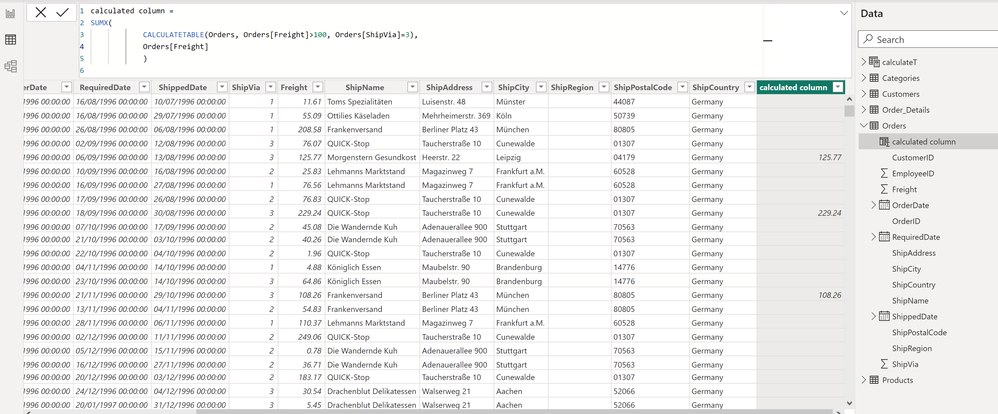cancel
Showing results for
Did you mean:Frequent Visitor

## SUMX with CALCULATETABLE inside

Hello,

I understand context transition happens when we have calculate/calculatetable or a named measure inside an interator. For example, if I create a calculated column and enter this formula =CALCULATE(SUMX(Table1, Table1[Field1]*Table1[Field2]) then I know the current row in the calculated column gets passed on as filter when the SUMX scans Table1 and we should see different values in each row in the calculated column.

However, I don't understand what exactly happens when we create a calculate column that contains a CALCULATETABLE inside SUMX? For example, this is my formula inside the calculated column:

calculated column=SUMX(

CALCULATETABLE(Orders, Orders[Freight]>100, Orders[ShipVia]=3),

Orders[Freight])
)

This formula then creates the below output. As you can see, we have distinct values appearing only in rows where the Freight > 100 and the ShipVia = 3.I was expecting to see repeated values in all rows because the SUMX is not wrapped around CALCULATE. For example, when we have something like calculated column = SUMX(Products, Products[Price]*Products[Qty]) we get the same repeated values down the column but if we wrap this formula inside CALCULATE we get a unique value for each row. So the question is, why does my above formula when its not wrapped inside a CALCULATE is still giving distinct values only for when Frieght > 100 and ShipVia=3.

1 ACCEPTED SOLUTIONSuper User

CALCULATETABLE is forcing context transition, so the current row essentially becomes the filter context. The filters on Freight and Ship Via then get merged into that context, so you are only seeing values for rows which match the Freight and Ship Via conditions. It is equivalent to writing

``````calculated column =
IF ( Orders[Freight] > 100 && Orders[ShipVia] = 3, Orders[Freight] )
``````
2 REPLIES 2Frequent Visitor

Cheers!Super User

CALCULATETABLE is forcing context transition, so the current row essentially becomes the filter context. The filters on Freight and Ship Via then get merged into that context, so you are only seeing values for rows which match the Freight and Ship Via conditions. It is equivalent to writing

``````calculated column =
IF ( Orders[Freight] > 100 && Orders[ShipVia] = 3, Orders[Freight] )
``````Announcements#### Exclusive opportunity for Women!

Join us for a free, hands-on Microsoft workshop led by women trainers for women where you will learn how to build a Dashboard in a Day!#### Power Platform Conference-Power BI and Fabric Sessions

Join us Oct 1 - 6 in Las Vegas for the Microsoft Power Platform Conference.Top Solution Authors
Top Kudoed Authors
Users online (1,869)